##The conic dual to the circumcircle

This is a particular case of the duality studied in TriangleProjectivitiesPlay.html . The conic c under consideration is the dual inscribed to triangle ABC, when the circumscribed conic coincides with the circumcircle. Several facts are related to this conic, which is no other than the Brocard ellipse of the triangle. Some of these facts are listed below.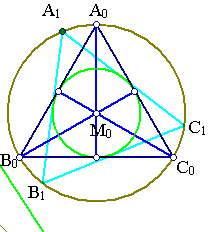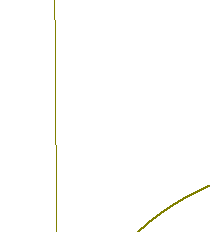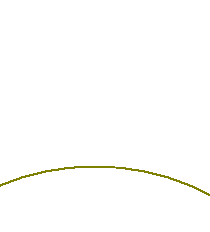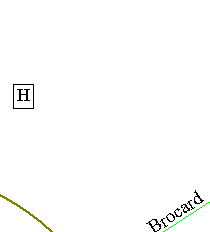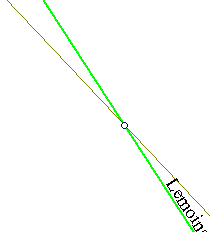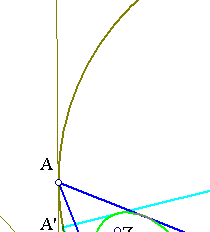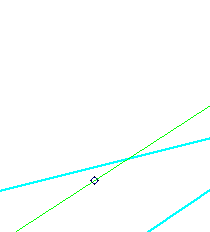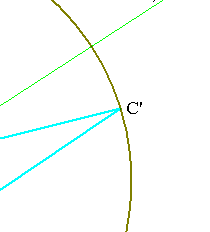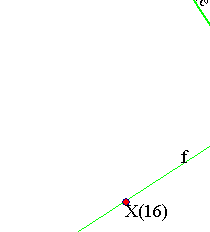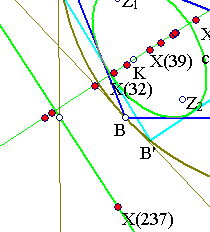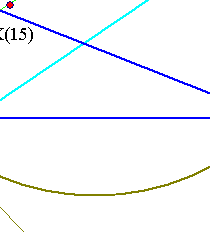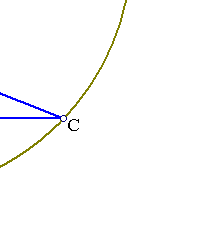Conic c can be obtained as the image of the incircle of the equilateral A0B0C0 through the projectivity H mapping the vertices A0, B0, C0 to A, B, C correspondingly and the center M0 of the equilateral to the symmedian or Lemoine point K of triangle ABC. These requirements completely determine H, which then maps the incircle of the equilateral to an ellipse inscribed in the triangle known as Brocard ellipse of the triangle. The point named x in the cited reference is here the symmedian point K and the corresponding trilinear polar is denoted here by L and coincides with the Lemoine axis of the triangle.
 The conic c is always an ellipse. Its focal points Z1, Z2 are the Brocard points of the triangle. Their line is parallel to L and its center is on the Brocard axis f joining K to the circumcenter O of ABC.
 Line f is orthogonal to L.
 Line L carries, among others, one other remarkable triangle-center namely X(237), the point where the Euler line of ABC meets the Lemoine axis. The trilinear polars of points of L are tangent to the ellipse.
 Line f carries several triangle centers. For example: the two isodynamic points X(15), X(16), the 3rd power point X(32), the Brocard midpoint X(39), X(52), X(58), X(61), X(62), X(182), X(284), X(371), X(372), X(389).
 Consider another equilateral A1B1C1 resulting by rotating A0B0C0 about its center. Then apply H to get triangle A'B'C'. This triangle shares with ABC several geometric characteristics such as:
[6.1] Same circumcircle and Brocard ellipse, same symmedian point, circumcenter O, lines f and L.
[6.2] Triangle centers of A'B'C' lying on f either remain the same or move on line f.
[6.3] Triangle centers of A'B'C' lying on L either remain fixed or move on line L.
 The incenters of A'B'C' describe another conic c', not belonging to the family generated by the incircle and its conjugate conic c, sharing though with c one axis.

CrossRatio.html
CrossRatio0.html
CrossRatioLines.html
GoodParametrization.html
Harmonic.html
Harmonic_Bundle.html
HomographicRelation.html
HomographicRelationExample.html
IncircleConjugate.html
Pascal.html
PascalOnTriangles.html
ProjectivityFixingVertices.html
Steiner_Ellipse.html
TriangleCircumconics.html
TriangleCircumconics2.html
TriangleProjectivitiesPlay.html
TrilinearPolar.html

 Produced with EucliDraw© http://users.math.uoc.gr/~pamfilos/